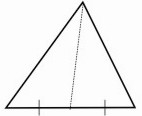HOME MATH DICTIONARY DOWNLOAD FEEDBACK DISCLAIMER
 Question: What do you mean by Median of a Triangle ? Answer: A median of a triangle is a line joining a vertex to the mid-point of the opposite side. A triangle has three medians which always meet at a point. This point is one-third of the way up each median and is called the centroid of the triangle.Example of Median of a Triangle: